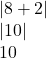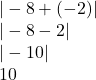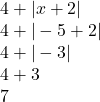Can anyone please answer these? Who ever answers correctly will be marked brainliest. 1. Find the absolute value of |8 + y| when

Question

1. Find the absolute value of |8 + y| when y = 2

2. Find the absolute value of |-8 + y| when y = -2

3. Simplify: 4 + |x + 2| when x = -5​

in progress 0
5 months 2021-08-17T02:09:35+00:00 2 Answers 0 views 0

1)10

2)10

3) 7

Step-by-step explanation:

Modulus means we always take the positive value for example

|-3| = +3

1) Find the absolute value of |8 + y| when y = 22) Find the absolute value of |-8 + y| when y = -23) Simplify 4 + |x + 2| when x = -5